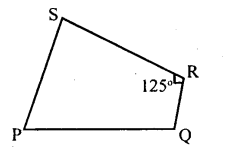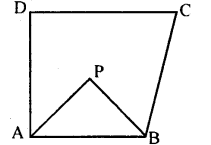# KSEEB Solutions for Class 8 Maths Chapter 15 Quadrilaterals Ex 15.1

In this chapter, we provide KSEEB SSLC Class 8 Maths Chapter 15 Quadrilaterals Ex 15.1 for English medium students, Which will very helpful for every student in their exams. Students can download the latest KSEEB SSLC Class 8 Maths Chapter 15 Quadrilaterals Ex 15.1 pdf, free KSEEB SSLC Class 8 Maths Chapter 15 Quadrilaterals Ex 15.1 pdf download. Now you will get step by step solution to each question.

### Karnataka State Syllabus Class 8 Maths Chapter 15 Quadrilaterals Ex 15.1

Question 1.
Two angles of a quadrilateral are 70° and 130° and the other two angles are equal. Find the measure of these two angles.
Let the equal angles be x
70 + 130 + x + x = 360°
[Sum of the angles of a quadrilateral 360°]
200 + 2x = 360°
2x = 160
x =$\frac { 160 }{ 2 }$
x = 80°
Each of the other two angles is 80°

Question 2.
In the figure. Suppose ∠P and ∠Q are supplementary angles and ∠R = 125 °. Find the measure of∠S.∠P + ∠Q +∠R + ∠s = 360°
[Sum of the angles of a quadrilateral]
180 + 125° + ∠S = 360°
[∠P and ∠Q are supplementary]
305 + ∠S = 360°
∠ S = 360 – 305
∠S = 55°

Question 3.
Three angles of a quadrilateral are in the ratio 2:3:5 and the fourth angles are 90°. Find the measure of the other three angles.
Three angles are in the ratio 2 : 3 : 5 Let the angles be 2x, 3x and 5x
[Sum of the angles of a quadrilateral]
2x + 3x + 5x + 90° = 360°
10x + 90 = 360
10x = 360 – 90
10x = 270°
x =$\frac { 270 }{ 10 }$ = 27°
∴ 2x = 2 × 27 = 54°
3x = 3 × 27 = 81°
5x = 5 × 27 = 135°
Three angle are 54°, 81° & 135°

Question 4.
In the adjoining figure, ABCD is a quadrilateral such that ∠D + ∠C = 100°. The bisectors of ∠A and ∠B meet at P.
Determine ∠APB.∠A + ∠B + ∠C + ∠D = 360°
[Sum of the angles of a quadrilateral]
∠A + ∠B = 360 – 100
∠A + ∠B = 260$\frac { 1 }{ 2 }$ ∠A +$\frac { 1 }{ 2 }$  ∠B =$\frac { 1 }{ 2 }$ × 260°
[Multiplying by$\frac { 1 }{ 2 }$ ]
∠PAB + ∠PBA = 130°
[AP and BP are bisectors of ∠A and ∠B]
In ∆ APB. ∠APB +∠PAB +∠PB A = 180°
∠PAB + ∠PBA + ∠APB = 180°
[Sum of the angles of a triangle]
∠APB + 130 = 180°
∠APB = 180 – 130
∠ APB = 50°

All Chapter KSEEB Solutions For Class 8 maths

—————————————————————————–

All Subject KSEEB Solutions For Class 8

*************************************************

I think you got complete solutions for this chapter. If You have any queries regarding this chapter, please comment on the below section our subject teacher will answer you. We tried our best to give complete solutions so you got good marks in your exam.

If these solutions have helped you, you can also share kseebsolutionsfor.com to your friends.

Best of Luck!!